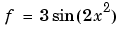MATLAB Function Referenceinline

Construct an inline object

Syntax

• ```g = inline(expr)
g = inline(expr,arg1,arg2,...)
g = inline(expr,n)
```

Description

```inline(expr) ``` constructs an inline function object from the MATLAB expression contained in the string `expr`. The input argument to the inline function is automatically determined by searching `expr` for an isolated lower case alphabetic character, other than `i` or `j`, that is not part of a word formed from several alphabetic characters. If no such character exists, `x` is used. If the character is not unique, the one closest to `x` is used. If two characters are found, the one later in the alphabet is chosen.

```inline(expr,arg1,arg2, ...) ``` constructs an inline function whose input arguments are specified by the strings `arg1`, `arg2`,`...`. Multicharacter symbol names may be used.

```inline(expr,n) ``` where `n` is a scalar, constructs an inline function whose input arguments are `x`, `P1`, `P2`, ... .

Remarks

Three commands related to `inline` allow you to examine an inline function object and determine how it was created.

`char(fun) `converts the inline function into a character array. This is identical to `formula`(`fun`).

`argname`s(`fun`) returns the names of the input arguments of the inline object `fun` as a cell array of strings.

`formula`(`fun`) returns the formula for the inline object `fun`.

A fourth command `vectorize`(`fun`) inserts a `.` before any` ^`, `*` or `/`' in the formula for `fun`. The result is a vectorized version of the inline function.

Examples

Example 1. This example creates a simple inline function to square a number.

• ```g = inline('t^2')
g =

Inline function:
g(t) = t^2
```

You can convert the result to a string using the `char` function.

• ```char(g)

ans =

t^2
```

Example 2. This example creates an inline function to represent the formula. The resulting inline function can be evaluated with the `argnames` and `formula` functions.

• ```f = inline('3*sin(2*x.^2)')

f =
Inline function:
f(x) = 3*sin(2*x.^2)

argnames(f)

ans =
'x'

formula(f)
ans =

3*sin(2*x.^2)ans =
```

Example 3. This call to `inline` defines the function `f` to be dependent on two variables, `alpha` and `x`:

• ```f = inline('sin(alpha*x)')

f =
Inline function:
f(alpha,x) = sin(alpha*x)
```

If `inline` does not return the desired function variables or if the function variables are in the wrong order, you can specify the desired variables explicitly with the `inline` argument list.

• ```g = inline('sin(alpha*x)','x','alpha')

g =

Inline function:
g(x,alpha) = sin(alpha*x)
```

© 1994-2005 The MathWorks, Inc.